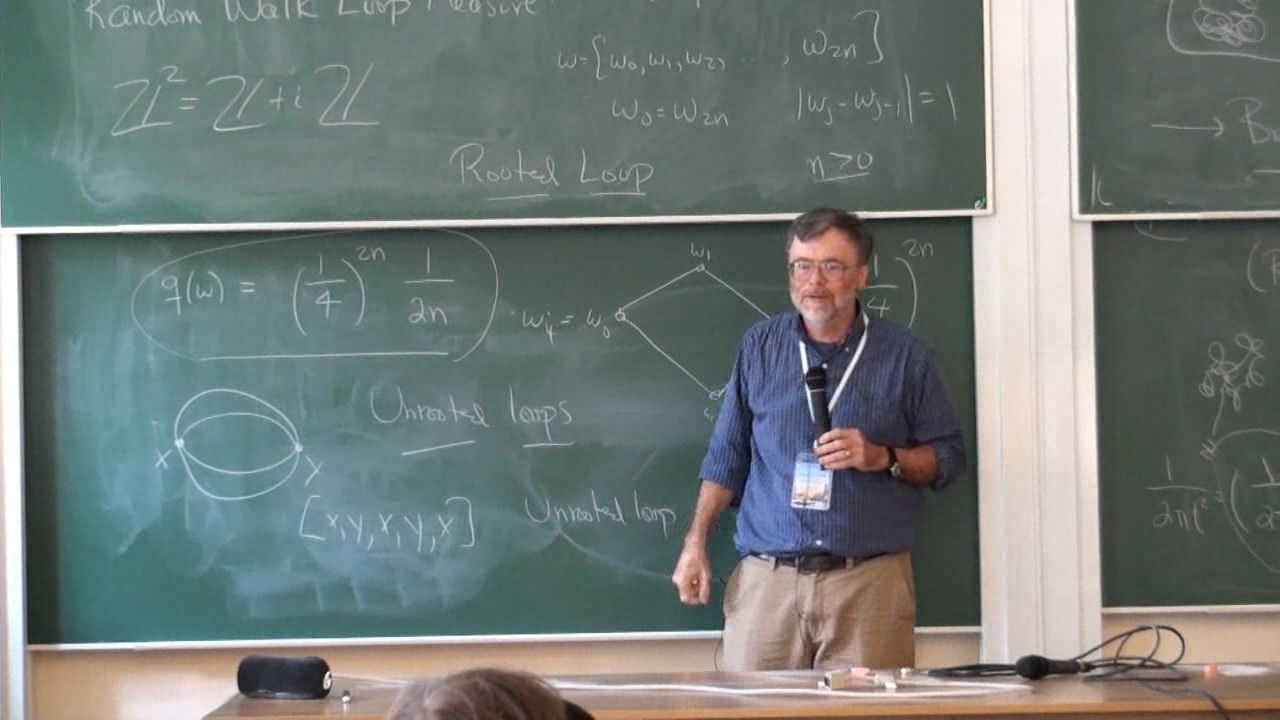# An introduction to the Schramm-Loewner Evolution

Курс
Предмет:
Лектор:The Schramm-Loewner evolution as orginally defined by Oded Schramm is a conformally invariant family of probability of measures connecting points on simply connected domains. I will give an introduction taking a "partition function" approach to these curves where the measures need not be probability measures. The first lecture will be a survey of what is known while the second will concentrate on the mathematical tools to prove results.

Курс прочитан в рамках St. Petersburg School in Probability and Statistical Physics

Источник информации

2